数学不好也能学会AI编程！微软亚洲研究院AI实践课程上新

10 月 9 日 微软研究院AI头条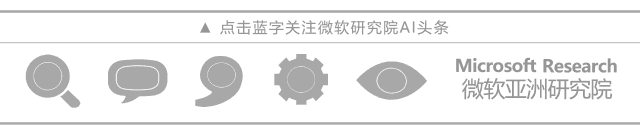• 从简单的代码入手，逐步教会学生神经网络的原理，每一步都有代码展现原理
• 有实训平台，可以在上面用 GPU 训练 AI 模型
• 有众多有趣+实用的案例
• 初步反响很好，程序员喜欢通过写代码来学习 AI，而不是推导公式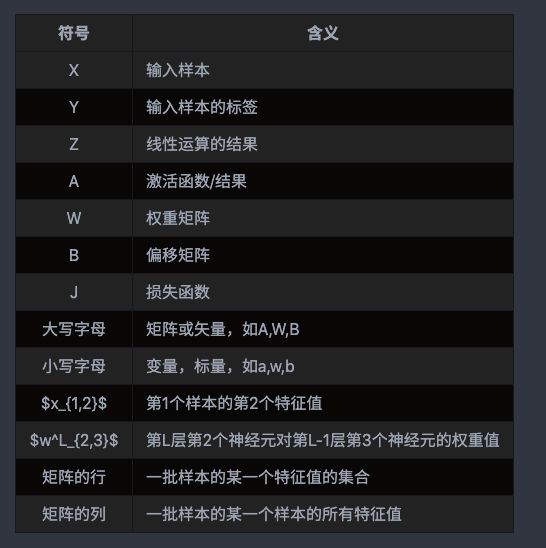• Windows 10 version 1809
• Visual Studio 2017 Community or above
• Python 3.6.6
• Jupyter Notebook (可选)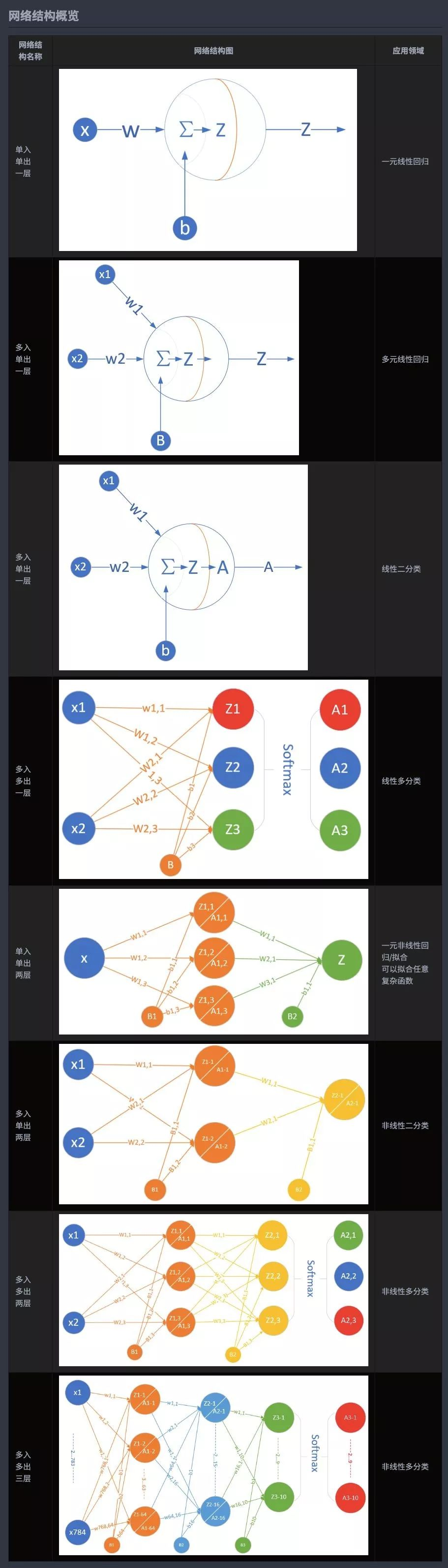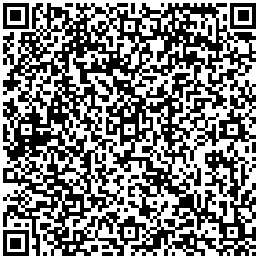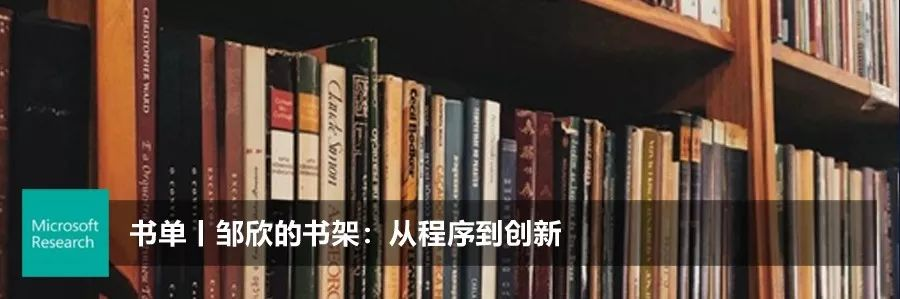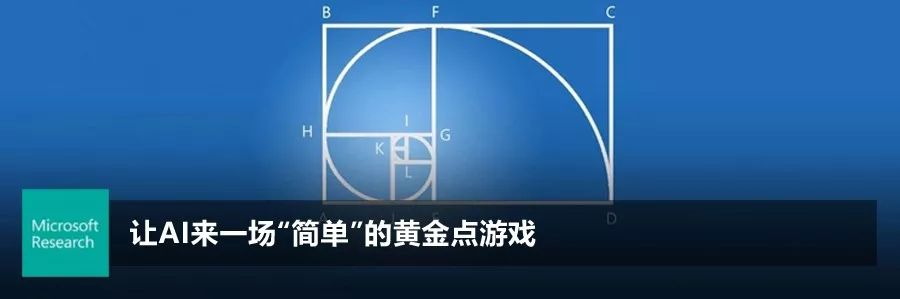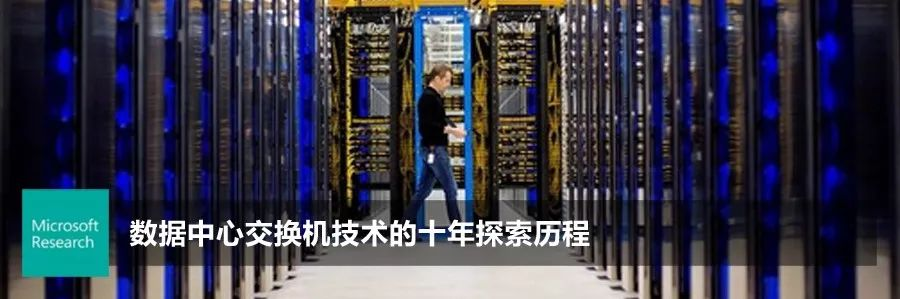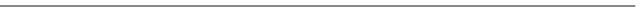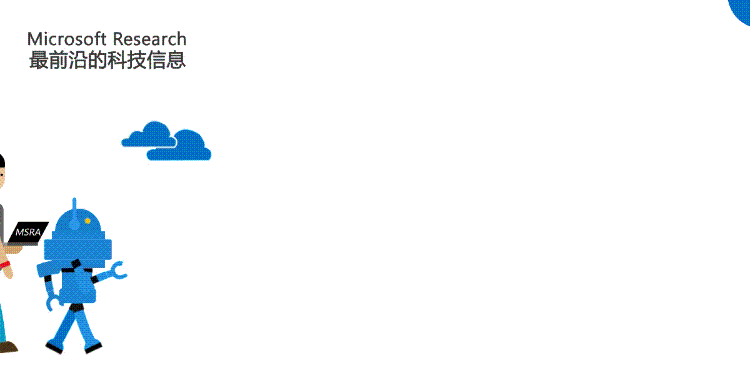0+2+

相关内容Fundamentals of Python Programmingpythonbook.pdf0+0+0+0+1+0+0+2+0+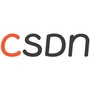0+0+0+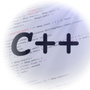0+0+0+0+4+0+0+1+0+0+1+0+0+0+0+0+0+0+
Top